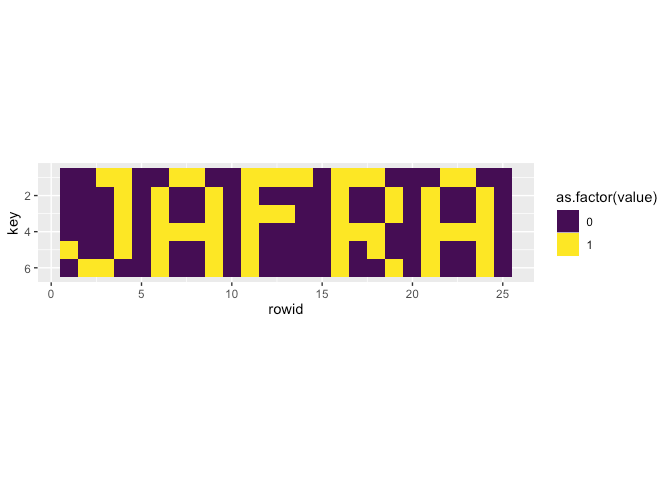Want to share your content on R-bloggers? click here if you have a blog, or here if you don't.

Solving Advent of Code 2019-08 with R and JavaScript.

[Disclaimer] Obviously, this post contains a big spoiler about Advent of Code, as it gives solutions for solving day 8.

The JavaScript code has been written in the same RMarkdown as the R code. It runs thanks to the `{bubble}` package: https://github.com/ColinFay/bubble

## R solution

### Part one

```library(magrittr)
library(purrr)

ipt <- strsplit(ipt, "")[] %>% as.numeric()

layers_size <- 6 * 25
l <- list()

for (i in 1: (length(ipt)/layers_size)){
l[[i]] <- ipt[1:150]
ipt <- ipt[151:length(ipt)]
}

mn <- l %>%
lapply(table) %>%
map_dbl("0") %>%
which.min()

l[[mn]] %>%
table()

## .
##   0   1   2
##   7  14 129

14 * 129

##  1806
```

### Part two

```v <- c()
for (i in seq_len(layers_size)){
idx <- map_dbl(l, i)
v[i] <- idx[idx %in% c(0,1)]
}

library(dplyr)
library(tidyr)
library(ggplot2)
library(tibble)
matrix(v, ncol = 6) %>%
as.data.frame() %>%
rowid_to_column() %>%
gather(key = key, value = value, V1:V6) %>%
mutate(key = gsub("V(.)", "\\1", key) %>% as.numeric()) %>%
ggplot(aes(rowid, key, fill = as.factor(value))) +
geom_tile() +
coord_fixed() +
scale_fill_viridis_d() +
scale_y_reverse()
```## JS solution

```var ipt = fs.readFileSync("input8.txt", 'utf8').split("").filter(x => x.length != 0 & x != '\n').map(x => parseInt(x));

var layers_size = 6 * 25;
var layer_n = ipt.length / layers_size;
var res = [];

function table(vec){
var tbl = {};
vec.map(function(x){
if (tbl[x]){
tbl[x] = tbl[x] + 1;
} else {
tbl[x] = 1;
}
})
return tbl;
}

for (var i = 0; i < layer_n; i ++){
res[i] = ipt.splice(0, layers_size);
}

var res_b = res.map(x => table(x));
var minim = Math.min.apply(Math, res_b.map(x => x['0']));
var smallest = res_b.filter(x => x['0'] == minim);

smallest["1"] * smallest["2"];

## 1806

var v = [];

for (var i = 0; i < layers_size; i ++){
var idx = res.map(x => x[i]);
v[i] = idx.find(z => z== 0 | z == 1);
}

var nn = [];

for (var i = 0; i < 6; i ++){
nn[i] = v.splice(0, 25).join(" ").replace(/0/g, " ");
}

nn

## [ '    1 1     1 1     1 1 1 1   1 1 1       1 1    ',
##   '      1   1     1   1         1     1   1     1  ',
##   '      1   1     1   1 1 1     1     1   1     1  ',
##   '      1   1 1 1 1   1         1 1 1     1 1 1 1  ',
##   '1     1   1     1   1         1   1     1     1  ',
##   '  1 1     1     1   1         1     1   1     1  ' ]
```